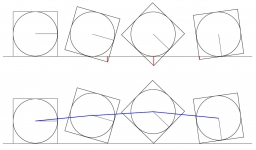# Square to rectangle

What is the ratio of the area of a square of side x to the area of a rectangle of a rectangle of width 2 x and length 3

Result

r = (Correct answer is: x/6)### Step-by-step explanation:Did you find an error or inaccuracy? Feel free to write us. Thank you!Tips to related online calculators
Check out our ratio calculator.

#### You need to know the following knowledge to solve this word math problem:

We encourage you to watch this tutorial video on this math problem:

## Related math problems and questions:

• Square vs rectangleSquare and rectangle have the same area contents. The length of the rectangle is 9 greater and width 6 less than side of the square. Calculate the side of a square.
• The circumferenceThe circumference and width of the rectangle are in a ratio of 5: 1. its area is 216cm2. What is its length?
• Rectangle 35Find the rectangle area when the diagonal is equal to 30 cms and the width is double the length.
• Two bodiesThe rectangle with dimensions 8 cm and 4 cm is rotated 360º first around the longer side to form the first body. Then, we similarly rotate the rectangle around the shorter side b to form a second body. Determine the ratio of surfaces of the first and seco
• Rectangle 3-4-5The sides of the rectangle are in a ratio of 3:4. The length of the rectangle diagonal is 20 cm. Calculate the content of the rectangle.
• RectangleThe length of the rectangle are in the ratio 5:12 and the circumference is 238 cm. Calculate the length of the diagonal and area of rectangle.
• Squares ratioThe first square has a side length of a = 6 cm. The second square has a circumference of 6 dm. Calculate the proportions of the perimeters and the proportions of the contents of these squares? (Write the ratio in the basic form). (Perimeter = 4 * a, conte
• Rectangle diagonalsIt is given a rectangle with an area of 24 cm2 a circumference of 20 cm. The length of one side is 2 cm larger than the length of the second side. Calculate the length of the diagonal. Length and width are yet expressed in natural numbers.
• A rectangle 2A rectangle has a diagonal length of 74cm. Its side lengths are in ratio 5:3. Find its side lengths.
• RectangleCalculate area of the rectangle if its length is 12 cm longer than its width and length is equal to the square of its width.
• Squares above sidesTwo squares are constructed on two sides of the ABC triangle. The square area above the BC side is 25 cm2. The height vc to the side AB is 3 cm long. The heel P of height vc divides the AB side in a 2: 1 ratio. The AC side is longer than the BC side. Calc
• HallRectangular hall will have pave by square tiles with a side length 15 cm. The hall has length 18 meters and width 3 m. How many tiles need to buy if 2 percent of the amount is disrupted during the work?
• RectangleThe length of the rectangle is 12 cm greater than 3 times its width. What dimensions and area this rectangle has if ts circumference is 104 cm.
• The sidesThe sides of the rectangle are in a ratio of 3: 5 and its circumference measures 72 cm. Calculate: a) the size of both sides of the rectangle b) the area of the rectangle c) the length of the diagonals
• PlotThe length of the rectangle is 8, smaller than three times the width. If we increase the width by 5% of the length and the length is reduced by 14% of the width, the circumference of the rectangle will be increased by 30 m. What are the dimensions of the
• Rectangle areaThe length of a rectangle of x units is increased by 10%, and its width of y units is increased by 15%. What is the ratio of the area of the old rectangle to the area of the new rectangle?
• The widthThe width of a rectangular garden is 4 m less than the length. If the area of a rectangular garden is 96 square meters, what is the dimension of the garden?